Reach Us+447482877761
Chemical Oscillations and Spatial Structures in Polymerisation Reactions | OMICS International
Chemical Sciences Journal
All submissions of the EM system will be redirected to Online Manuscript Submission System. Authors are requested to submit articles directly to Online Manuscript Submission System of respective journal.

# Chemical Oscillations and Spatial Structures in Polymerisation Reactions

Issa Katime1*, Juan A Pérez–Ortiz1 and Eduardo Mendizábal2

1Grupo de Nuevos Materiales, Departamento de Química Física, Facultad de Ciencia y Tecnología, Campus de Leioa, Spain

Corresponding Author:

Issa Katime
Grupo de Nuevos Materiales
Departamento de Química Física
Facultad de Ciencia y Tecnología
Campus de Leioa, Spain
E-mail: [email protected]

Received Date: September 11, 2015 Accepted Date: September 15, 2015 Published Date: September 22, 2015

Citation: Katime I, Pérez–Ortiz JA, Mendizábal E (2015) Chemical Oscillations and Spatial Structures in Polymerisation Reactions. Chem Sci J 6:108. doi:10.4172/2150-3494.1000108

Copyright: © 2015 Katime I, et al. This is an open-access article distributed under the terms of the Creative Commons Attribution License, which permits unrestricted use, distribution, and reproduction in any medium, provided the original author and source are credited.

Visit for more related articles at Chemical Sciences Journal

#### Abstract

This article discusses the possibility of coupling a polymerization reaction to an oscillatory kinetic model, complemented by diffusion which can lead to spatial structure. We used three well–known mathematical models of oscillators: a variant of the Rossler multivibrator, a model proposed by Edelstein, and the Oregon Oscillator. One or some of the terms in the equations of these models come from a polymerization reaction, while the other terms of these equations will come from collateral processes. So, almost any reaction could become oscillatory and/or with dissipative structure, adding the adequate collateral processes. The propagation stages are considered as invariants and initiation reactions of order α = 0, 1, or 2, and termination reactions of order β = 1 or 2 are assumed. Except in the case α = 1, β = 2, all six remaining reactions combinations (α, β) can be coupled to least one, and often to several of the models. The effects of destabilization a stable homogeneous steady state by the presence of diffusion is also discussed, which is always be possible.

#### Keywords

Reaction kinetics; Rossler multivibrador; Oregon oscillator; Oscillatory chemical reactions

#### Introduction

Theoretical and experimental studies of oscillating reactions have been studied at many laboratories and the interest of this type of phenomena has increased rapidly which led to the discovery of oscillations in biochemical and chemical systems . Interestingly, in the early studies, thermodynamicists and applied mathematicians worked out models for such reactions and discussed the feasibility of oscillations in homogeneous chemical systems. Later a general criterion was available for the type of chemical reactions that could present undamped oscillations .

When the first discoveries in oscillatory chemical reactions  and spatial structures were found in chemical systems  (e.g., concentration waves), mathematical expressions to fit experimental results were looked for.

Adequate models for the chemical oscillations  are provided by non-linear ordinary differential equations when they are considered as precursors of the formal kinetic development. Partial differential equations  could explain the appearance of spatial rearrangements when they are applied to the reaction kinetics considering spatial diffusion. There are some kinetic reaction mechanisms, which are in general very complex that can be fit to mathematical models to predict the apparition ofoscillationsand/or spatial arrangements . Recently, it has been suggested  that the mathematical equations of these models could be divided in two groups. Some of these equations will consider the main reactions (object to study), and the rest of them will take in account collateral processes. So, almost any reaction could become oscillatory and/or with dissipative structure by adding the adequate collateral processes. In this paper, examples of the application of this idea to polymerisation reactions, is shown.

#### Materials and Methods

Polymerisation processes and rate equations 

Three steps are considered in the polymerisation reactions:

Initiation: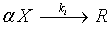where reaction rate is of the form Ri∼ Xα, and α can take the values 0, 1 or 2

Propagation: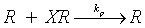reaction rate is of the form Rp ∼ RX

Termination: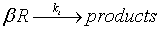In this case reaction rate is of the form Rt ∼ Rβ and β can take the values 1 or 2.

By assuming the steady state for the propagators R, that is Ri = Rt =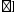Rt, it is obtained that R ∼ Xα/β; then the expression for the polymerisation rate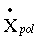becomes: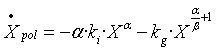(1)

where kg includes the constants kp and kt.

The term X represents monomer concentration, and the propagation stages are considered as invariants and the different mechanisms of the polymerisations will be denoted using the symbols [α] [7,8], where the initiation reaction (α) can be of zero, first, 1, or second order and the termination reaction (β) can be of of first or second order.

Analysis without diffusion

Ordinary differential equations system [4,9] in two variables, X and Y, will be considered(2)

where the variable Y represents the concentration of a specie that appears in a collateral process. The steady states denoted by SS(i), fulfill the equalities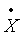=0 ,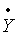=0 and the X and Y concentrations can be calculated by solving M(X0,Y0) = 0, N(X0,Y0) = 0 equations. A linearized variational system [10,11] is obtained when considering the perturbations defined as: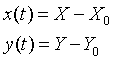(3)

the system is of the form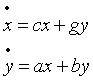(4)

where the coefficients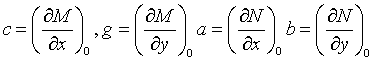are the elements of the characteristic no–diffusive matrix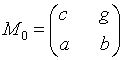This matrix trace is

T0 = b + c (5)

and its determinant is

Det0 = bc – a (6)

In the neighbourhood of the steady state (SS) the solutions of (3) are in the form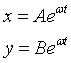(7)

where, ω are the roots of the equation:

ω2 – T0·ω + Det0 = 0 (8)

The steady state is unstable if, at least, one of these roots has a positive real part. It occurs when Det0 < 0. However, this condition causes the SS to be a “saddle point”, which normally involves explosions, and so, it is discarded as realistic chemical model.

A root with a positive real part can be obtained if T0 > 0.

If T0 = 0 and the discriminant is:

Δ0 = T0 2 – 4Det0 (9)

if Det0 < 0 then Δ0 > 0, the steady state is a node; if Det0 > 0 then Δ0 < 0, i the steady state s a focus.

In all the equations systems that will be examined, the condition Det0 > 0 will be fulfilled, and the transitions from T0< 0 to T0 = 0 and to T0> 0 will be possible, that is, from a stable focus (T0 ≤ 0, Δ0 ≈ – 4Det0< 0) to node (T0 = 0, Δ0 = – 4Det0 < 0) and to unstable focus (T0 ≥ 0, Δ0≈ – 4Det0 < 0). Hopf  proved that this bifurcation leads to the apparition of a limit cycle around the unstable focus, with sustained oscillations of the system. In this paper, the Hopf bifurcations existence in all the studied cases is verified.

Including the diffusion process

If diffusion effects along one dimension (r) are considered, the equations (2) take the form [13,10]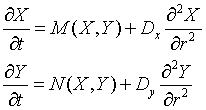(10)

where Dx and Dy are diffusion coefficients, respectively. In this context, the steady states are named homogeneous steady state (HSS). Their stability can also be studied by linearization, and when suitable perturbations for the desired boundary conditions are defined. In this paper, the Neuman´s conditions  (of no–flow in the system boundary) are used: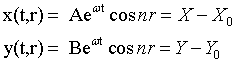(11)

where n is the wave number, which under the conditions x(0, L) = ± A and y(0, L) = ±B, must satisfy,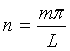where L is the length of the system and m = 0, 1, 2, 3, .....(an integer) The secular matrix of the linearized system takes the form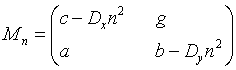(12)

whose trace is

Tn= T0 – (Dx+ Dy)·n2 (13)

and the determinant is

Detn= Det0 – n2·(bDx+ cDy) + Dx·Dy·n4 (14)

Now, trace and determinant depend on the wave number, n. The roots of the secular equation

ω2 – Tn·ω + Detn = 0 (15)

will be also dependent on the wave number. As in the no diffusive case, if one of those roots has a positive real part, the homogeneous steady state will be unstable. That can be so because of the conditions Detn < 0, or Tn > 0. The condition Tn > 0 is impossible to be fulfilled, unless in the no diffusive case T0 > 0, that is, the homogeneous steady state was already unstable in diffusion absence. So, it is impossible a homogeneous stable steady state, which fulfilled T0 < 0, Det0 > 0, to be destabilized by diffusion. Besides, in the case T0 > 0, if ω had a positive real part because of being Tn > 0, that positive real part would be ωRe+ = Tn/2 if Δn = Tn 2 – 4Detn < 0, or ωRe+ = [(Tn + Δn1/2)/2] if Δn ≥ 0; in one or the other case, the higher positive value of this real part will be reached when n = 0. That means that in the case of where are several solutions (for some n values), when it is allowed by the limit conditions, the homogeneous solution (n = 0), will be amplified faster and predominates above the no homogeneous ones, and the presence of spatial structures is not probable.

The condition Detn < 0 has not been considered yet. If p = n2, it can be expressed as a second grade polynomial:

Detn = Det0 – p·(bDx+ cDy) + DxDyp2 < 0 (16)

and it is possible that it could be fulfilled in some interval p1 < p < p2 (although T0 > 0 and Det0 > 0, case in which it can be said that the homogeneous steady state can be destabilized by diffusion. Independently of p2> p1> 0, and p1p2 = Det0/Dx·Dy, it is necessary Det0 > 0 to be firstly fulfilled. Moreover, as p1 + p2 = [(b·Dx + c·Dy)/Dx·Dy] > 0, it is necessary that bDx+ cDy > 0. Finally, the condition for that interval to exist is that the discriminant must be positive,

Δ(16) = (b·Dx+ c·Dy)2 – 4Dx·Dy·Det0 > 0 (17)

If all of those conditions are fulfilled, as Tn < T0 < 0, Detn< 0 < Det0, the inequality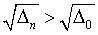will be also fulfilled, and so,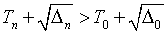could be accepted. The positive real part of ω will be higher for some n ≠ 0, than for n = 0, and if that is so, the no homogeneous perturbation increases more quickly than the homogeneous one (if both of them coexist and compete).

If the homogeneous steady state is unstabilized for any wave number, n, an initial fluctuation having a Fourier component with that n, will force the system to go out from the homogeneous steady state and a new spatial arrangement produced by fluctuations  will be obtained.

Now, the three mathematical models will be analysed.

#### Models

Rossler modified model [7,15,16]

1A. without diffusion: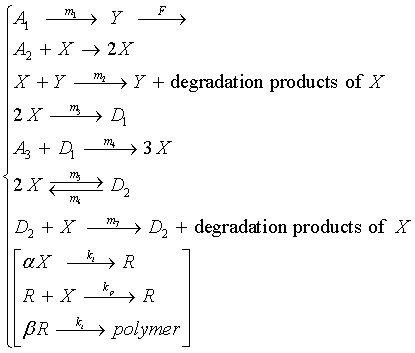The kinetic equations: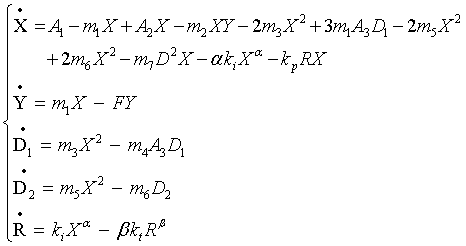When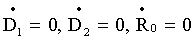, they are reduced to two equations: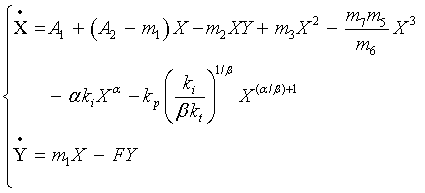and the following equations are obtained: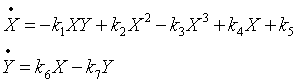(18)

where the values of k1, k2, k3, k4, k5, k6 and k7 are defined as follows: Considering the polymerizations types {0, 1} and {0, 2}: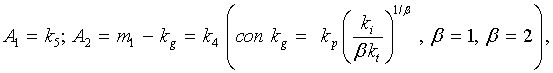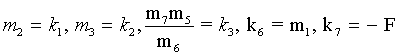for the polymerization type {1, 1}: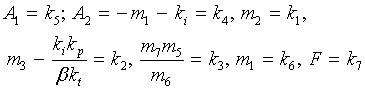for polymerization type {2, 1}: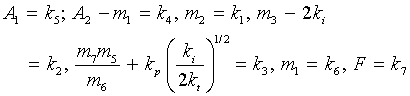when the polymerization type {2, 2} is considered: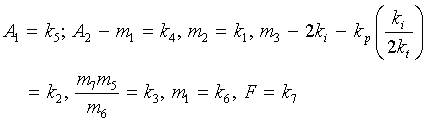A. A concrete case  will be considered. With the following values of the constants: k1 = 1, k2 = 0.9, k3 = 1, k4 = 0.2, k5 = 0.01, k6 = 1.5ε and k7 = ε. The system becomes :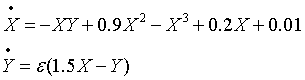(19)

This system has an only one homogeneous steady state (X0 = 0.271, Y0 = 0.4065) and its secular matrix is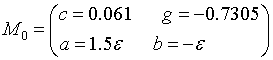with T0 = 0.061 – ε, will be positive if ε < 0.061, and Det0 = 0.3455ε > 0. So, (19) admits Hopf bifurcation and a limit cycle around the unstable steady state for ε < 0.061.

1B. Considering that there is a diffusion process, (18) becomes: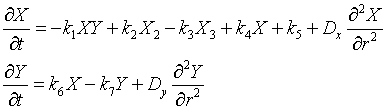(20)

and using the constants values of Section 1A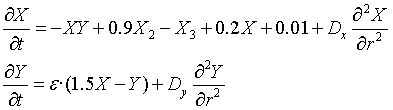(21)

The secular matrix is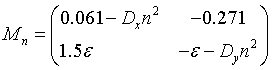whose trace is Tn = 0,061 – ε – (Dx+ Dy)n2, and the determinant, Detn= 0.3455ε – n2·(0.061·Dy – ε·Dx) + DxDyn4. The condition Det0> 0 is fulfilled; the bDx + cDy > 0 implies that 0.061Dy > ε·Dx, and (17) requires (0.061Dy – εDx)2> 4Dx·Dy·0.3455ε. For ε = 1 (value in which T0 < 0), Dx = 1, Dy = 600 (adequate units), this condition is also fulfilled. p = 0.025 could be a possible value of p that makes Detn< 0; for that p value, the real part of T0 is (0.021/2), and its higher than the real part of T0, – (T0/2) < 0 (since Δ0≈ – 0.5 < 0, the homogeneous steady state was an stable state).

Edelstein model [7,17,18]

2A. This model is based in an enzymatic mechanism [18-20] whose substrate is monomer X. That makes possible to be applied in biochemical polymerisations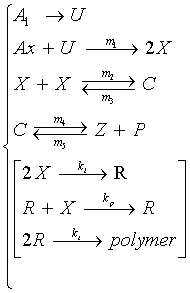The kinetic equations are:Which are reduced to two variables if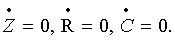Observe that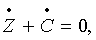then Z + C = B = constant, resulting in the following equations: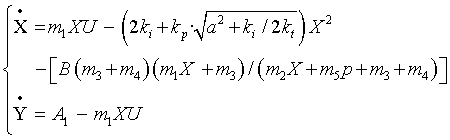with the definitions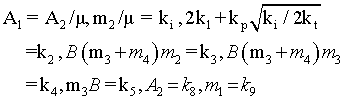it is obtained: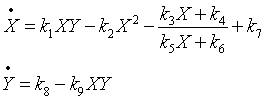(30)

This model applies only to the polymerization type {2,2}.

Considering the following values  for the constants (in adequate units): k1 = 1/μ, k2 = 1/μ, k3 = 60/μ, k4 = 60/μ, k5 = 1, k6 = 2.2, k7 = 30/μ, k8 = 16.858 and k9 = 1, the equations system becomes: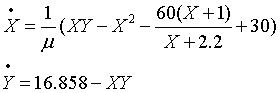(31)

which has a steady state in X0 = 2, Y0 = 8.429. The secular matrix is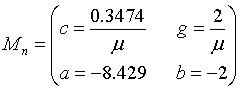and the trace,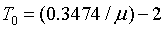, if T0 = 0.1737, then T0 > 0. Moreover, Det0 = (16.1633/ T0) > 0. So, if μ < 0.1737, a limit cycle around the unstable steady state exits.

2B. If the diffusion is considered, (30) can be written as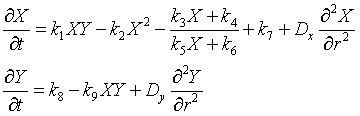(32)

and with the values for the parameters previously used: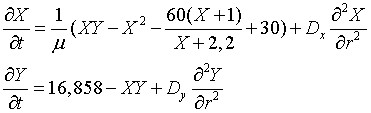(33)

The secular matrix is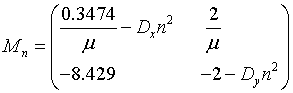whose trace is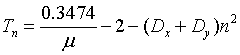and the determinant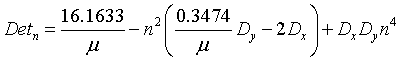Det0 > 0 is fulfilled. In order to fulfill the conditions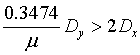and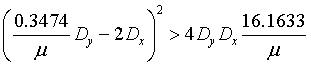the values μ = 1 (that leads to T0< 0), Dx = 1, Dy = 600, are used. So, the conditions for Detn < 0 are fulfilled if p = 0.2, value that provides a real part of ω (0.0181/2), higher than the real part of ω0 (without diffusion), (ω0/2) < 0, since Δ0 < 0. The homogeneous steady state was a stable focus and it could be destabilized by diffusion.

Oregon oscillator [7,21], Stiffly coupled oregonator 

5A. It was conceived first by Field and Noyes  as a model developed from the well-known Belousov–Zhabotinsky oscillating reaction . Those authors explained it in three variables, but it is possible to reduce it to two variables assuming that the third one is always maintained in steady state (stiff coupling) , without losing its main important characteristics.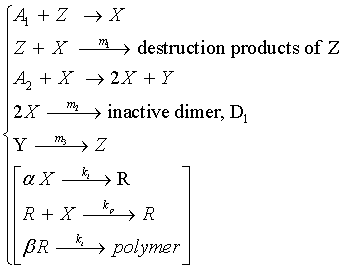The kinetic equations are: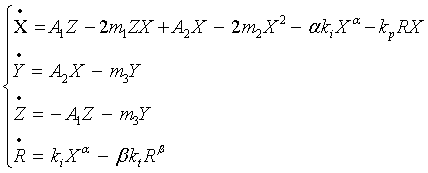Which are reduced to two variables if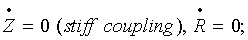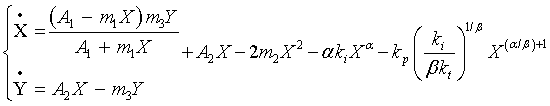Considering polymerizations type {0, 1} and {0,2} and with the definitions: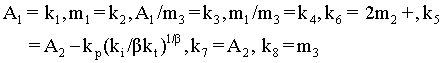The kinetic equations take the form: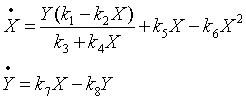(34)

Polymerization type {1, 1} with the definitions: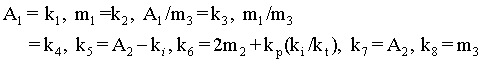results in (34).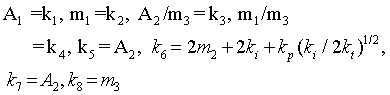Polymerization {2, 2} and the definitions:

give as a result equations (34).

3A. Assuming the following values for the parameters  k1 = 1, k2 = 1, k3 = 1, k4 = 1, k5 = 1 and k6 = 8.375.10–6 (proposed value by Field y Noyes considering the bromine chemistry , in order to explain the Belousov–Zhabotinsky reaction); k7 = ε and k8 = ε, the system (34) becomes: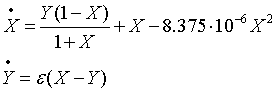(35)

There is a steady state in X0 = Y0 = 488.68. The secular matrix is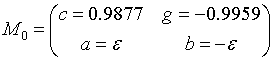and the trace is T0 = 0.9877 – ε. Det0 = 8.176.10–3ε > 0. If ε < 0.9877, the steady state will be unstable and a limit cycle around it will be arise.

3B. Introducing the diffusion process, (34) can be written as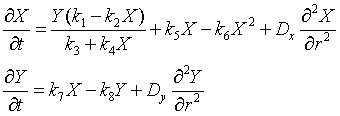(36)

Using the same constants that in 3B, the system is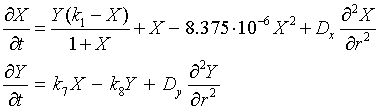(37)

with the following secular matrix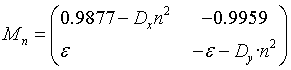whose trace is,

Tn = 0.9877 – ε – (Dx+ Dy)·n2

and the determinant,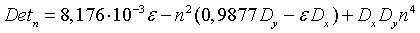When Det0 > 0, the conditions 0.9877·Dy > ε·Dx, and (17) if ε = 1 (that means T0 < 0), Dx = 1, Dy = 2, can be fulfilled; for example, the value p = 0.2 satisfies the conditions for Detn < 0. For that value, the real part of ωn is 0.2837/2, higher than the part corresponding to n = 0, that would be T0/2 < 0 (since Δ0 < 0). The homogeneous steady state is a stable focus.

#### Conclusion

Except for the polymerisation {1, 2}, the rest of the polymerisations types considered in this paper can fit, at least, to one and frequently to some of the three mentioned oscillators. Supercritical bifurcation defbpf, with limit cycle apparition in absence of diffusion, is possible in all the considered models. Moreover, in all cases, it is possible to get an unstable homogeneous steady state of diffusion, and a homogeneous steady state (stable in diffusion presence) can be destabilized by diffusion. By the moment, it is difficult to predict the scientific [25-30] or technological applications that could have the theoretical development made in this paper.

#### References

1. Prigogine I, Balescu R (1955) Bull CiSciAcad Roy Beig 41: 912.
2. Belousov BP (1959) Sb RefRadiatMed 147: 145
3. Zhabotinsky AM (1964) DoklAkadNauk USSR157: 392.
4. Bronson R (2008) Ecuaciones diferenciales, McGraw-Hill, México.
5. Fitzgibbon WE (1984)Partialdifferentialequations and dynamicalsystems.Pitman, London.
6. Thom R (1983) Mathematicalmodels of morphogenesis. Ellis Horwood Ltd.,Chichester.
7. Katime I, Pérez-Ortíz JA, Mendizábal E (2011) Simple models of oscillatingpolymerizations:Dimericformautocatalysis. ChemEngSci 66: 2261-2265.
8. Katime I, Pérez-Ortiz JA, Mendizábal E, Zuluaga F(2009) Harmonicoscillations in a polymerization. Intern J ChemKin41: 507-511.
9. Nicolis G, Portnow J (1973) Chemicaloscillations. ChemRev 73: 365-384.
10. Balslev I,Degn H (1974) Unifiedtheory of temporal and spatialinstabilities. Faraday SympChemSoc 9: 233-240.
11. Katime I, Pérez-Ortíz JA, Zuluaga F, Mendizábal E(2010) Minimummodels of damped and limitcycleoscillations in a polymerization. Chem EngSci65: 6292-6295.
12. Uppal A,Ray WH, Poore AB (1974) Onthedynamicbehavior of continuousstirredtankreactors. Chem Eng Sci 29: 967-985.
13. Poincaré H (1885) Ontheequilibrium of a mass of fluid given a rotationalmovement. Acta Math7: 259
14. Katime I, Pérez-Ortiz JA, Zuluaga F Mendizábal E(2008) Polybrusselator-typemodels. Int J ChemKin 40: 617-623.
15. Katime I, Pérez-Ortiz JA, Morales J, Mendizábal E (2014) ModelsforOscillatingPolymerization: ActivationbyProduct in a SystemwithFlow. Int J Chem Kin 46: 405-417.
16. Rossler OE(1974) Faraday SympChemSoc 9: 87
17. Rössler OE (1972) A principleforchemicalmultivibration.J TheorBiol 36: 413-417.
18. Gray BF, Aarons LJ (1974)Small parasiticparameters and chemicaloscillations. Faraday SympChemSoc 9: 129.
19. Edelstein BB (1970) Biochemicalmodelwithmultiplesteadystates and hysteresis.J TheorBiol 29: 57-62.
20. Edelstein BB (1970) Instabilitiesassociatedwithdissipativestructure.J TheorBiol 26: 227-241.
21. Tyson JJ, Light JC (1973) Properties of twoâ��componentbimolecular and trimolecularchemicalreactionsystems. J Chem Phys59: 4164.
22. Field RJ,Noyes RM(1974) Oscillations in chemicalsystems. IV. Limitcyclebehavior in a model of a real chemicalreaction. J Chem Phys 60: 1877.
23. Tyson JJ (1973) Somefurtherstudies of nonlinearoscillations in chemicalsystems. J Chem Phys58: 3919.
24. Field RJ, Noyes RM (1974) A modelillustratingamplification of perturbations in an excitable médium. Faraday SympChemSoc 9: 21-27.
25. Katime I, Pérez JA, Terrón A (1984) Introducción a la termodinámica de procesos irreversibles. Reacciones oscilantes. Servicio Editorial de la Universidad del País Vasco, Bilbao.
26. Lefever R (1974) Faraday SympChemSoc 9: 160.
27. Heinrichs M, Schneider FW (1981) Relaxationkinetics of steadystates in thecontinuousflowstirredtank reactor. Response tosmall and largeperturbations: criticalslowingdown. J ChemPhys 85: 2112-2116.
28. Kanamaru T (2007) Van der Pol oscillator. Scholarpedia 2: 2202
29. Tyson JJ (1975) Classification of instabilities in chemicalreactionsystems. J Chem Phys 62: 1010
30. Epstein IR, Pojman JA (1999) Overview: Nonlineardynamicsrelatedtopolymericsystems. Chaos 9: 255-259.
Select your language of interest to view the total content in your interested language

### Article Usage

• Total views: 12577
• [From(publication date):
December-2015 - Sep 18, 2019]
• Breakdown by view type
• HTML page views : 8670

## Post your commentCan't read the image? click here to refresh
###### Peer Reviewed Journals

Make the best use of Scientific Research and information from our 700 + peer reviewed, Open Access Journals

International Conferences 2019-20

Meet Inspiring Speakers and Experts at our 3000+ Global Annual Meetings

Top